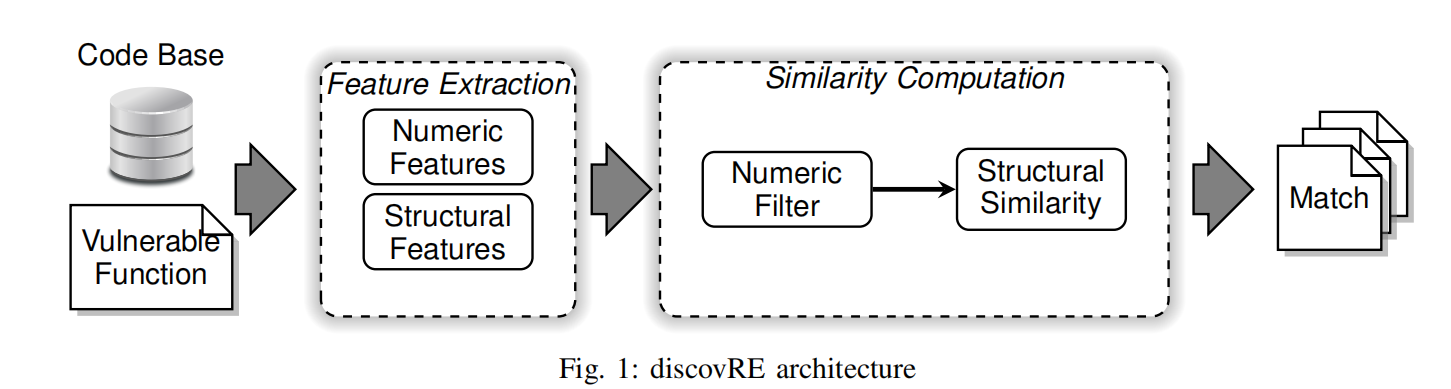# discovRE：Efficient Cross-Architecture Identification of Bugs in Binary Code阅读笔记

## 二进制代码相似性检测

Posted by Yunlongs on March 20, 2020

## discovRE: Efficient Cross-Architecture Identification of Bugs in Binary Code

### 方法概述输入：IDA反汇编过的二进制函数

Numeric Filter：把输入函数当作search pattern，使用KNN算法来基于数字特征寻找相似函数。
Structural Filter：基于maximum common subgraph isomorphism (MCS)建立Structural Similarity评估标准。是最复杂的filter。

### 方法实现

#### A. Data set

（Ⅰ）开源软件选择：要能够在不同的平台、编译器、操作系统运行。 尽可能的多覆盖各种库。
BitDHT, GnuPG, ImageMagick,LAME, OpenCV , SQlite and stunnel 。 总共包含超过31k个函数

（Ⅱ）函数去重： 重复的函数可能会造成跨平台的偏差。将所有引用内存地址的比特清零，然后计算比特校验码。
（Ⅲ）Identification of robust numeric features: 评估特征robustness的标准：1.仅在所有的编译选项下变化小。2.值的分布空间应当大。

#### B.Numeric filter

（1）数据标准化：因为不同的特征具有不同的范围，所以会导致在KNN计算距离时出现问题。所以每个值都采用均值为0，标准差为1来标准化。

（2）Evaluation of the numeric filter： 采用k-d trees作为距离测量标准，并使用PCA进行降维

#### C. Structural Similarity

$G_{f}:=(\lbrace v | v \text { is basic block in } f\rbrace,\lbrace(u, v) | u \text { redirects to } v\rbrace)$

（1）Basic block distance：在这里，我们不仅仅使用函数的结构属性，我们还要额外的使用各个基本块中各自的特征。例如：

$d_{B B}=\frac{\sum \alpha_{i}\left|c_{i f}-c_{i g}\right|}{\sum \alpha_{i} \max \left(c_{i f}, c_{i g}\right)}$

（2）Graph matching algorithm: 这里基于最大公共子图同构问题(MCS)来计算图的相似性。

$d_{\text {mes.orig}}\left(G_{1}, G_{2}\right):=1-\frac{\left|m c s\left(G_{1}, G_{2}\right)\right|}{\max \left(\left|G_{1}\right|,\left|G_{2}\right|\right)}$

$d_{m c s}\left(G_{1}, G_{2}\right):=1-\frac{\left|m c s\left(G_{1}, G_{2}\right)\right|-\sum d_{B B}\left(b_{i}, b_{j}\right)}{\max \left(\left|G_{1}\right|,\left|G_{2}\right|\right)}$

。。。。后面跳过一大段部分无关紧要的算法对比，参数选择等部分。

### EVALUATION

#### A. Similarity Metric

（1）随机选择要搜寻的目标函数$f_n^{c1,a1}$
（2）随机选择一个匹配的函数$f_n^{c2,a2}$
（3）随机选择一个有99，999个函数的集合$F$
（4）构建代码库$C=F \cup\left\lbrace f_{n}^{c 2, a 2}\right\rbrace$，$(|C|=100,000)$